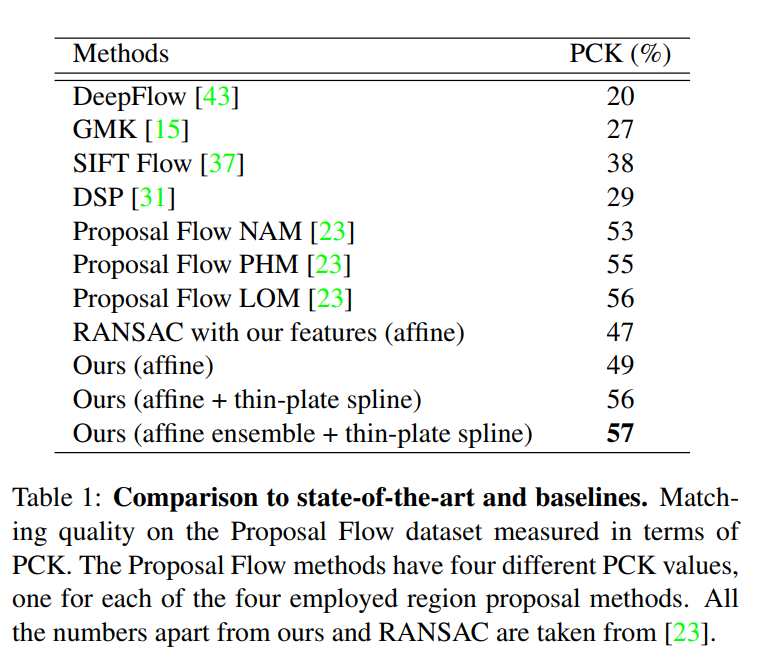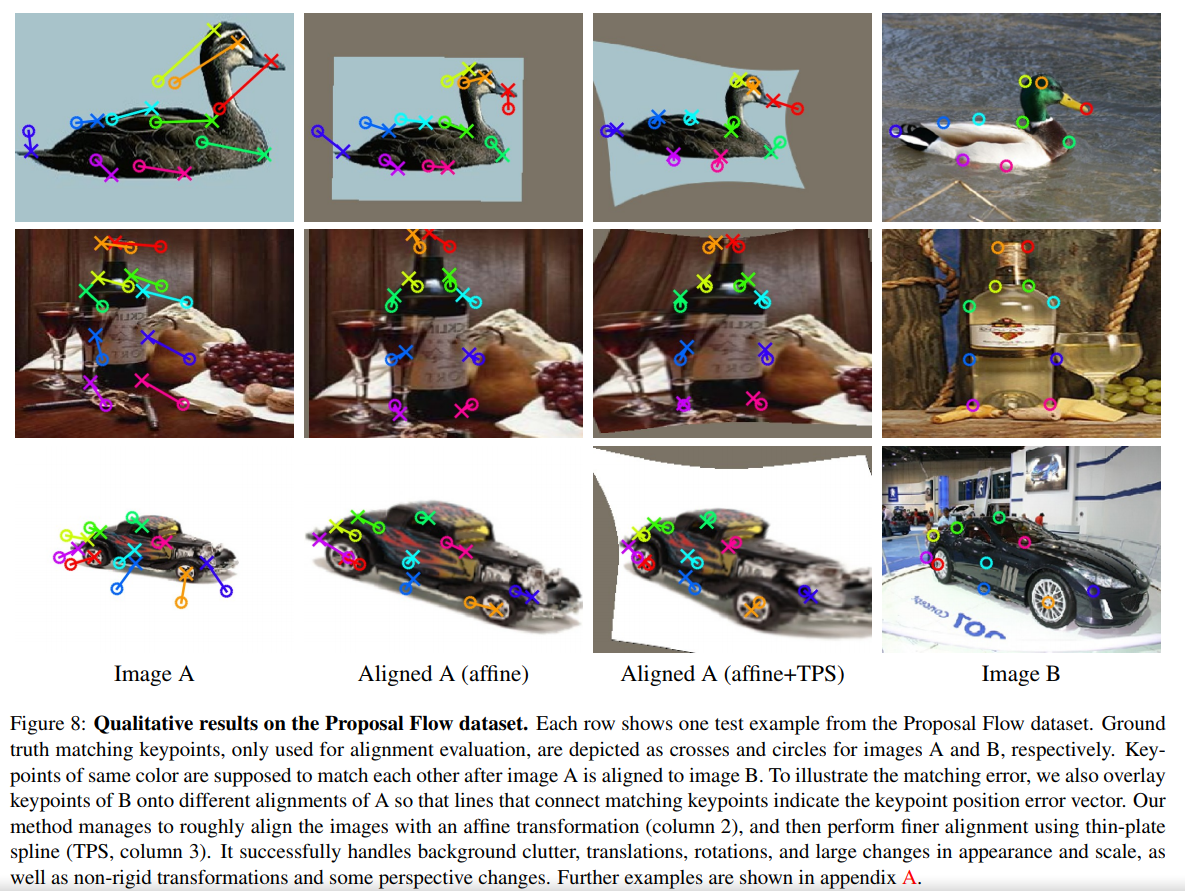## Summary

The authors propose a convolutional neural network architecture for geometric matching, trainable end-to-end. They also show that the model is trainable using synthetically warped images without needing any manual annotation.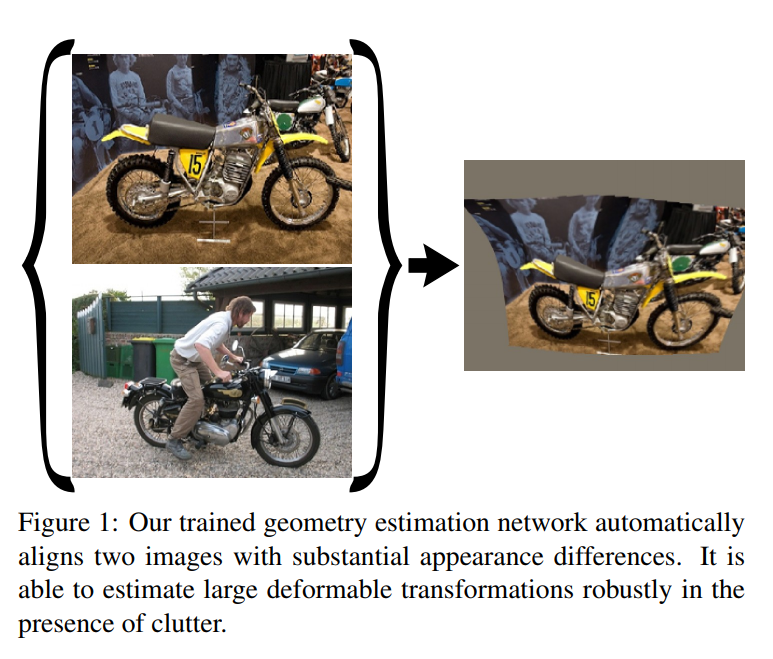### Model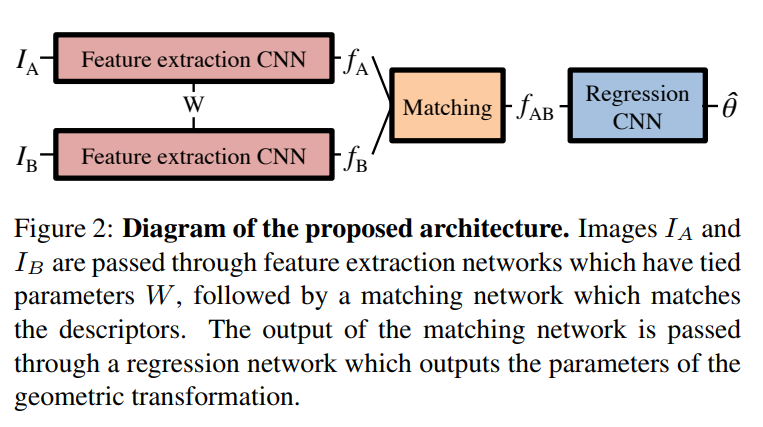The proposed model is divided into three steps:

1. Extracting features from the two input images using siamese networks (CNN)
2. Matching features by computing all pairwise similarities
3. Predicting the geometric transformation parameters using a regression network (CNN)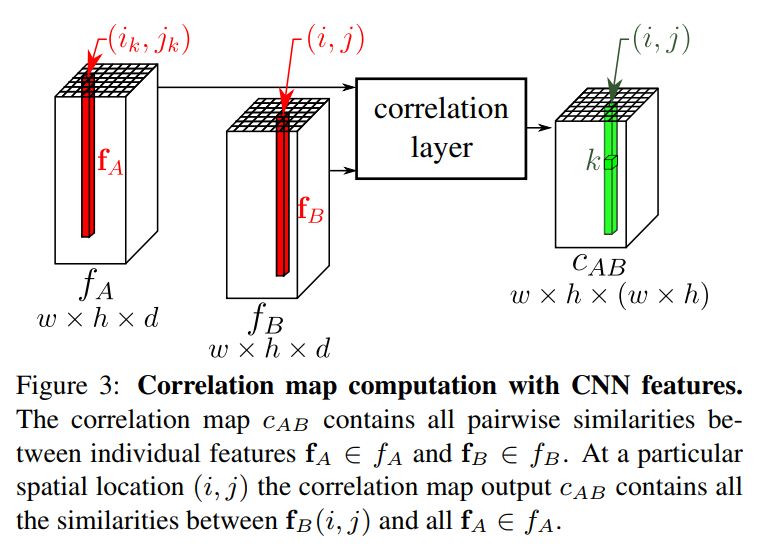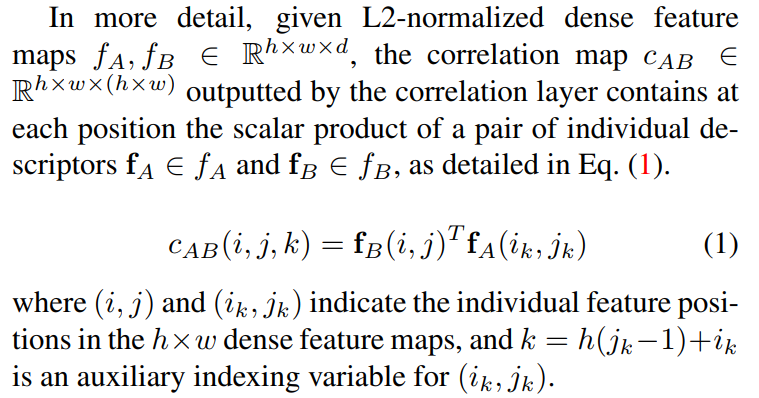### Model stacking

Instead of trying to directly predict a complex transformation, the authors propose to apply their model twice, starting with a simple linear transformation, then a more complex transformation:

1. Affine transformation (6 dof linear transformation = translation/rotation/non-isotropic scaling and shear)
2. Thin-plate spline transformation with 18 parameters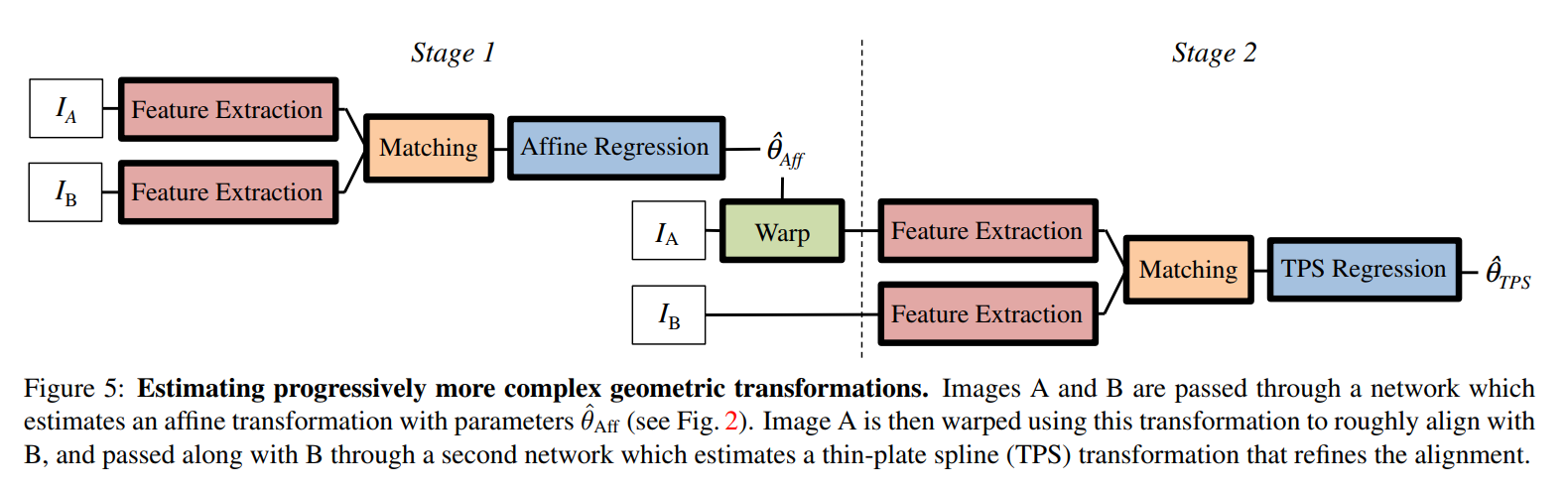### Training

The model is trained by measuring the loss on an “imaginary” grid of points which is deformed by the transformation. The loss is then the summed squared distances between the points deformed by the ground truth transformation and the predicted transformation.

Note that the affine transformation network and the TPS transformation network are trained independently.

### Synthetic image generation

The training dataset is automatically created from a public image dataset, by performing random transformations.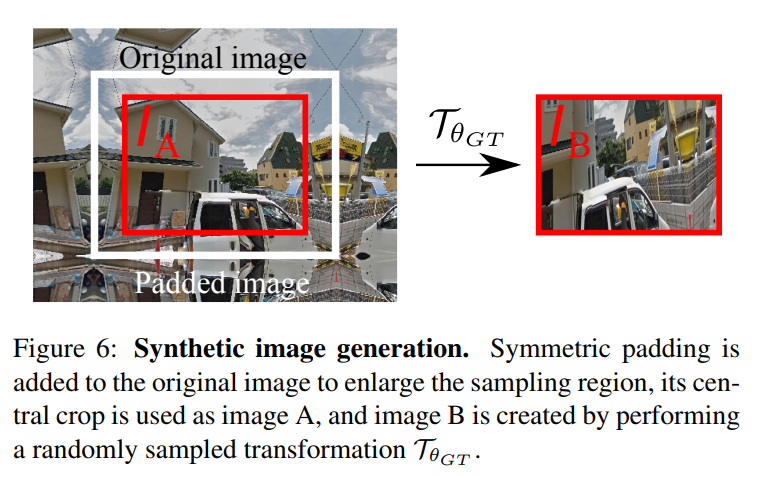## Experiments and Results

Training dataset:

• Tokyo Time Machine (40k Google Street View images of Tokyo)

Evaluation dataset:

• Proposal Flow (900 image pairs with large intra-class variations, e.g. ducks of different species, cars of different make, etc.)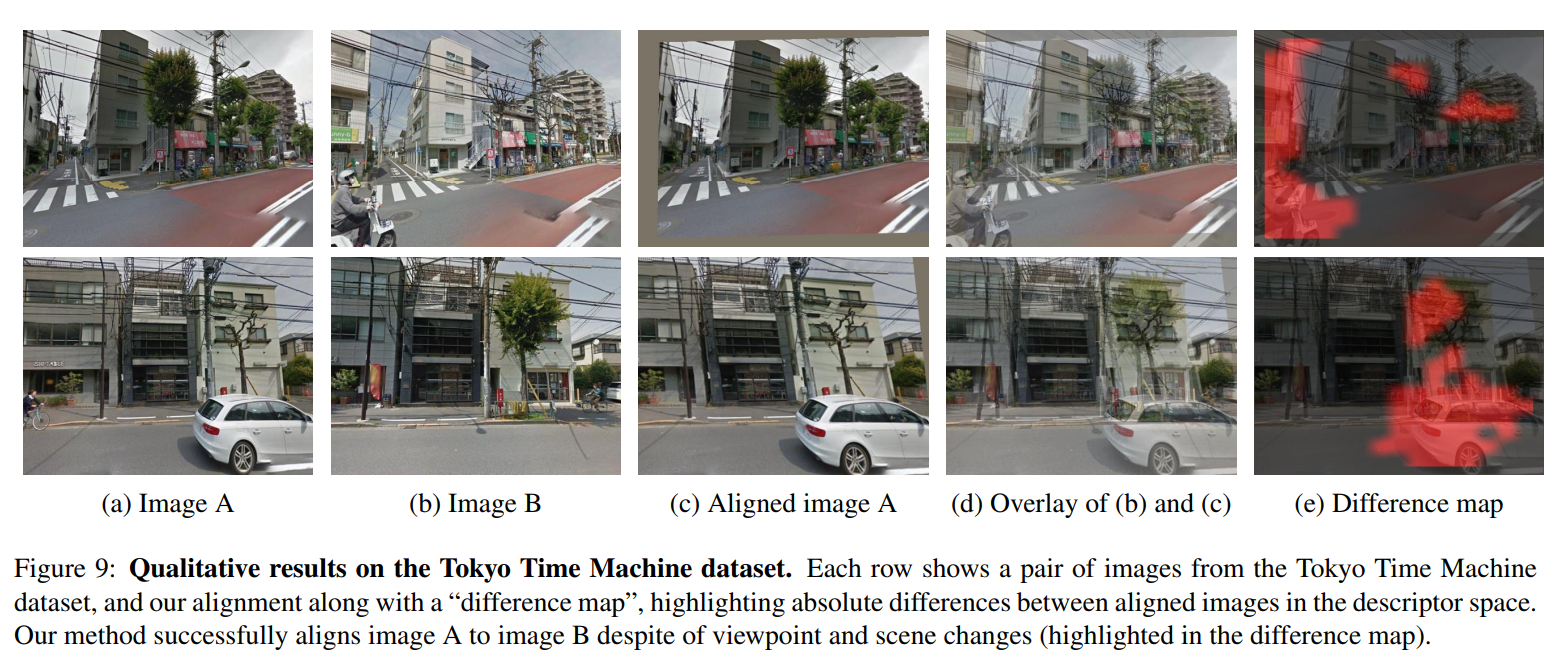The Proposal Flow task is to predict matching keypoint locations from an input image to a target image. The predictions are evaluated using average probability of correct keypoint (PCK). PCK is the proportion of keypoints that are correctly matched (i.e. within a certain distance of the target location).